Properties of Solids

As you should remember from the kinetic molecular theory, the molecules in solids are not moving in the same manner as those in liquids or gases. Solid molecules simply vibrate and rotate in place rather than move about. Solids are generally held together by ionic or strong covalent bonding, and the attractive forces between the atoms, ions, or molecules in solids are very strong. In fact, these forces are so strong that particles in a solid are held in fixed positions and have very little freedom of movement. Solids have definite shapes and definite volumes and are not compressible to any extent.

There are two main categories of solids—crystalline solids and amorphous solids. Crystalline solids are those in which the atoms, ions, or molecules that make up the solid exist in a regular, well-defined arrangement. The smallest repeating pattern of crystalline solids is known as the unit cell, and unit cells are like bricks in a wall—they are all identical and repeating. The other main type of solids are called the amorphous solids. Amorphous solids do not have much order in their structures. Though their molecules are close together and have little freedom to move, they are not arranged in a regular order as are those in crystalline solids. Common examples of this type of solid are glass and plastics.

There are four types of crystalline solids:

Ionic solids—Made up of positive and negative ions and held together by electrostatic attractions. They’re characterized by very high melting points and brittleness and are poor conductors in the solid state. An example of an ionic solid is table salt, NaCl.

Molecular solids—Made up of atoms or molecules held together by London dispersion forces, dipole-dipole forces, or hydrogen bonds. Characterized by low melting points and flexibility and are poor conductors. An example of a molecular solid is sucrose.

Covalent-network (also called atomic) solids—Made up of atoms connected by covalent bonds; the intermolecular forces are covalent bonds as well. Characterized as being very hard with very high melting points and being poor conductors. Examples of this type of solid are diamond and graphite, and the fullerenes. As you can see below, graphite has only 2-D hexagonal structure and therefore is not hard like diamond. The sheets of graphite are held together by only weak London forces!Metallic solids—Made up of metal atoms that are held together by metallic bonds. Characterized by high melting points, can range from soft and malleable to very hard, and are good conductors of electricity.

CRYSTAL STRUCTURES WITH CUBIC UNIT CELLS (From https://eee.uci.edu/programs/gchem/RDGcrystalstruct.pdf)

Crystalline solids are a three dimensional collection of individual atoms, ions, or whole molecules organized in repeating patterns. These atoms, ions, or molecules are called lattice points and are typically visualized as round spheres. The two dimensional layers of a solid are created by packing the lattice point “spheres” into square or closed packed arrays. (See Below).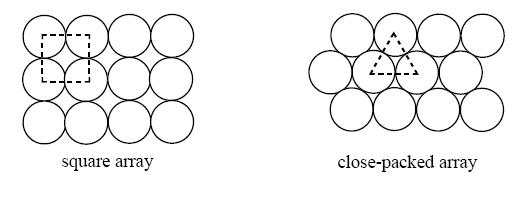Figure 1: Two possible arrangements for identical atoms in a 2-D structure

Stacking the two dimensional layers on top of each other creates a three dimensional lattice point arrangement represented by a unit cell. A unit cell is the smallest collectionof lattice points that can be repeated to create the crystalline solid. The solid can be envisioned as the result of the stacking a great number of unit cells together. The unit cell of a solid is determined by the type of layer (square or close packed), the way each successive layer is placed on the layer below, and the coordination number for each lattice point (the number of “spheres” touching the “sphere” of interest.)

Primitive (Simple) Cubic Structure

Placing a second square array layer directly over a first square array layer forms a "simple cubic" structure. The simple “cube” appearance of the resulting unit cell (Figure 3a) is the basis for the name of this three dimensional structure. This packing arrangement is often symbolized as "AA...", the letters refer to the repeating order of the layers, starting with the bottom layer. The coordination number of each lattice point is six. This becomes apparent when inspecting part of an adjacent unit cell (Figure 3b). The unit cell in Figure 3a appears to contain eight corner spheres, however, the total number of spheres within the unit cell is 1 (only 1/8th of each sphere is actually inside the unit cell). The remaining 7/8ths of each corner sphere resides in 7 adjacent unit cells.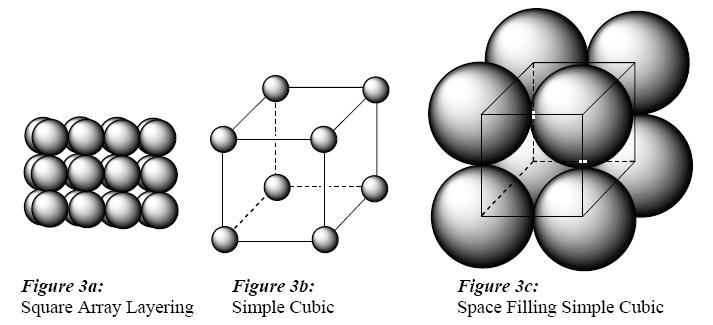The considerable space shown between the spheres in Figures 3b is misleading: lattice points in solids touch as shown in Figure 3c. For example, the distance between the centers of two adjacent metal atoms is equal to the sum of their radii. Refer again to Figure 3b and imagine the adjacent atoms are touching. The edge of the unit cell is then equal to 2r (where r = radius of the atom or ion) and the value of the face diagonal as a function of r can be found by applying Pythagorean’s theorem (a2 + b2 = c2) to the right triangle created by two edges and a face diagonal (Figure 4a). Reapplication of the theorem to another right triangle created by an edge, a face diagonal, and the body diagonal allows for the determination of the body diagonal as a function of r (Figure 4b).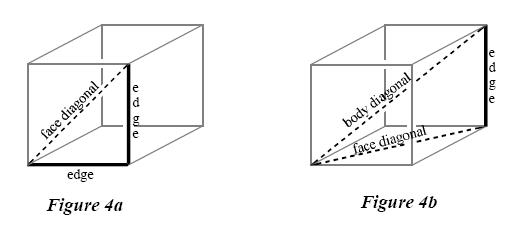Few metals adopt the simple cubic structure because of inefficient use of space. The density of a crystalline solid is related to its "percent packing efficiency". The packing efficiency of a simple cubic structure is only about 52%. (48% is empty space!)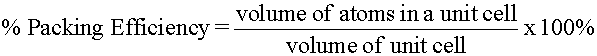Body Centered Cubic (bcc) Structure

A more efficiently packed cubic structure is the "body-centered cubic" (bcc). The first layer of a square array is expanded slightly in all directions. Then, the second layer is shifted so its spheres nestle in the spaces of the first layer (Figures 5a, b). This repeating order of the layers is often symbolized as "ABA...". Like Figure 3b, the considerable space shown between the spheres in Figure 5b is misleading: spheres are closely packed in bcc solids and touch along the body diagonal. The packing efficiency of the bcc structure is about 68%. The coordination number for an atom in the bcc structure is eight. How many total atoms are there in the unit cell for a bcc structure? Draw a diagonal line connecting the three atoms marked with an "x" in Figure 5b. Assuming the atoms marked "x" are the same size, tightly packed and touching, what is the value of this body diagonal as a function of r, the radius? Find the edge and volume of the cell as a function of r.Cubic Closest Packed (ccp)

A cubic closest packed (ccp) structure is created by layering close packed arrays. The spheres of the second layer nestle in half of the spaces of the first layer. The spheres of the third layer directly overlay the other half of the first layer spaces while nestling in half the spaces of the second layer. The repeating order of the layers is "ABC..." (Figures 6 & 7). The coordination number of an atom in the ccp structure is twelve (six nearest neighbors plus three atoms in layers above and below) and the packing efficiency is 74%.Figure 6: Close packed Array Layering. The 1st and 3rd layers are represented by light
spheres; the 2nd layer, dark spheres. The 2nd layer spheres nestle in the spaces of the 1st
layer marked with an “x”. The 3rd layer spheres nestle in the spaces of the 2nd layer that
directly overlay the spaces marked with a “·” in the 1st layer.Figure 7a & 7b: Two views of the Cubic Close Packed Structure

If the cubic close packed structure is rotated by 45° the face centered cube (fcc) unit cell can be viewed (Figure 8). The fcc unit cell contains 8 corner atoms and an atom in each face. The face atoms are shared with an adjacent unit cell so each unit cell contains ½ a face atom. Atoms of the face centered cubic (fcc) unit cell touch across the face diagonal (Figure 9). What is the edge, face diagonal, body diagonal, and volume of a face centered cubic unit cell as a function of the radius?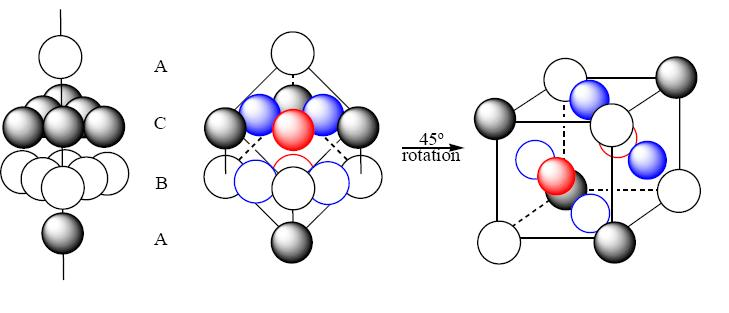Figure 8: The face centered cubic unit cell is drawn by cutting a diagonal plane through
an ABCA packing arrangement of the ccp structure. The unit cell has 4 atoms (1/8 of
each corner atom and ½ of each face atom).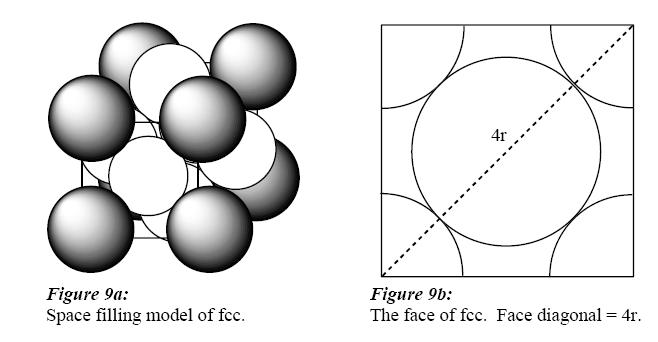Figure 9a:Space filling model of fcc. Figure 9b: The face of fcc. Face diagonal = 4r.

Ionic Solids

In ionic compounds, the larger ions become the lattice point “spheres” that are the framework of the unit cell. The smaller ions nestle into the depressions (the “holes”) between the larger ions. There are three types of holes: "cubic", "octahedral", and "tetrahedral". Cubic and octahedral holes occur in square array structures; tetrahedral and octahedral holes appear in close-packed array structures (Figure 10). Which is usually the larger ion – the cation or the anion? How can the periodic table be used to predict ion size? What is the coordination number of an ion in a tetrahedral hole? an octahedral hole? a cubic hole?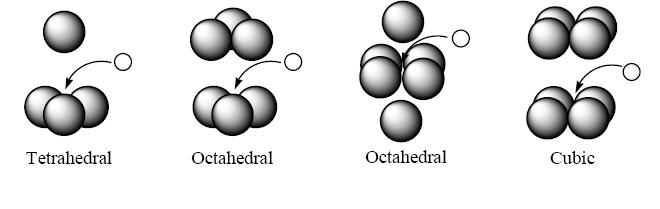Figure 10. Holes in ionic crystals are more like "dimples" or "depressions" between the
closely packed ions. Small ions can fit into these holes and are surrounded by larger ions
of opposite charge.

The type of hole formed in an ionic solid largely depends on the ratio of the smaller ion’s radius the larger ion’s radius (rsmaller/rlarger). (Table 1).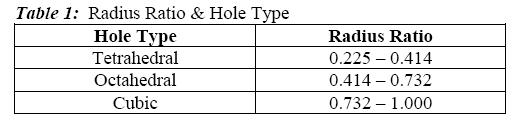Empirical Formula of an Ionic Solid

Two ways to determine the empirical formula of an ionic solid are: 1) from the number of each ion contained within 1 unit cell 2) from the ratio of the coordination numbers of the cations and anions in the solid.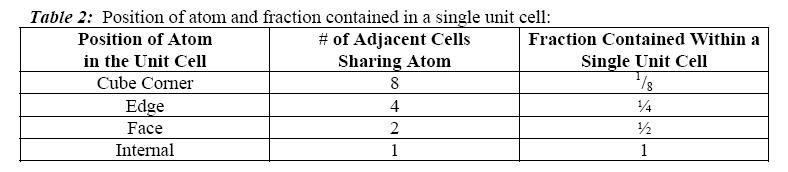Example: Find the empirical formula for the ionic compound shown in Figures 11 & 12.

First Method: When using the first method, remember most atoms in a unit cell are shared with other cells. Table 2 lists types of atoms and the fraction contained in the unit cell. The number of each ion in the unit cell is determined: 1/8 of each of the 8 corner X ions and 1/4 of each of the 12 edge Y ions are found within a single unit cell. Therefore, the cell contains 1 X ion (8/8 = 1) for every 3 Y ions (12/4 = 3) giving an empirical formula of XY3. Which is the cation? anion? When writing the formula of ionic solids, which comes first?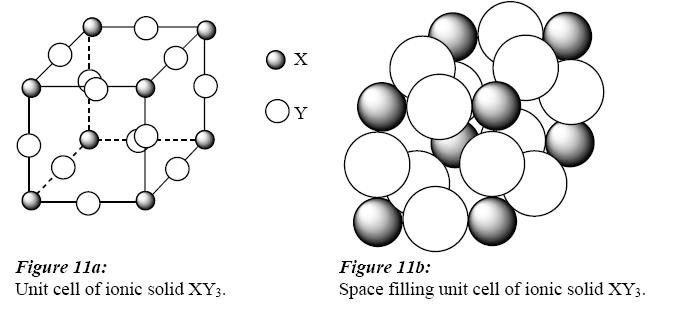Second Method: The second method is less reliable and requires the examination of the crystal structure to determine the number of cations surrounding an anion and vice versa. The structure must be expanded to include more unit cells. Figure 12 shows the same solid in Figure 11 expanded to four adjacent unit cells. Examination of the structure shows that there are 2 X ions coordinated to every Y ion and 6 Y ions surrounding every X ion. (An additional unit cell must be projected in front of the page to see the sixth Y ion ). A 2 to 6 ratio gives the same empirical formula, XY3.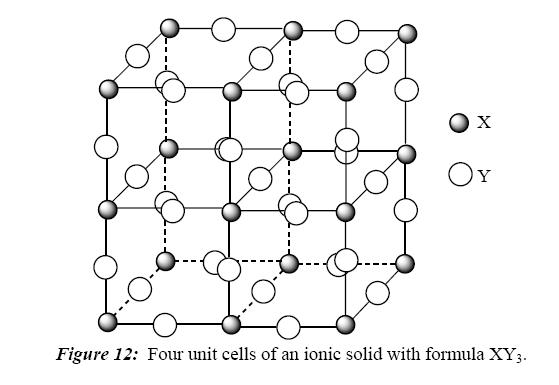Summary: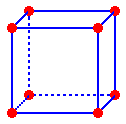Simple Cubic: 1 total atom per cell (1/8 each corner)Body Centered Cubic: 2 atoms per cell (1 in center and 1/8 for each corner)Face Centered Cubic: 4 atoms per cell
(1/2 per face and 1/8 for each corner)